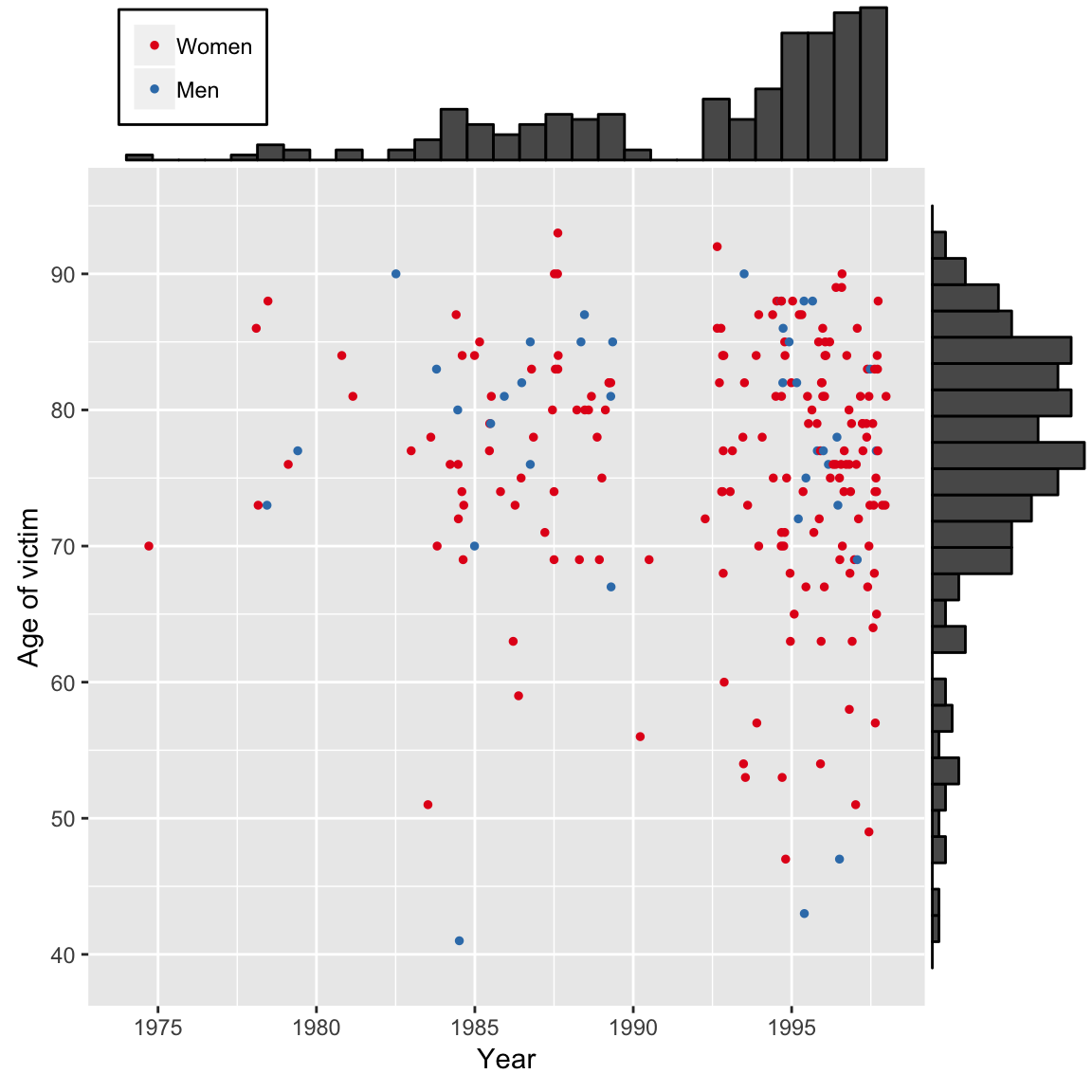Data are contained in 00-1-shipman-confirmed-victims-x.csv, and taken from Chronological List of Decided Cases in the Shipman Inquiry.

library(magrittr)
library(ggplot2)
library(ggpubr)
library(ggExtra)

# scatter-plot
s <- ggplot(df, aes(x=fractionalDeathYear, y=Age, colour=reorder(gender2,gender))) # initialise plot for the scatter-chart
s <- s + geom_point(size=1) # assign scatter chart-type with size 1 points
s <- s +  labs(x ="Year", y="Age of victim") # Adds axis labels
s <- s + scale_x_continuous(breaks=seq(1975, 1995, 5), limits = c(1974,1998)) #x-axis labels every 5 years and between 74 and 98
s <- s + scale_y_continuous(breaks=seq(40, 90, 10), limits = c(39,95)) # y-axis every 10 years and between 39 and 95
#s <- s  + scale_size_continuous(name = "Size", guide = FALSE)  # turns off size legend
s <- s + scale_colour_brewer(palette = "Set1") # sets the colour palette
s <- s + theme(legend.position=c(0.125,1.12 ), legend.background = element_rect(colour = "black"), legend.title = element_blank()) # positions. borders, and un-titles the legend

# with marginal histogram
ggMarginal(s, type="histogram")Figure 0.1 A scatter-plot showing the age and the year of death of Harold Shipman’s 215 confirmed victims. Bar-charts have been added on the axes to reveal the pattern of ages and the pattern of years in which he committed murders.